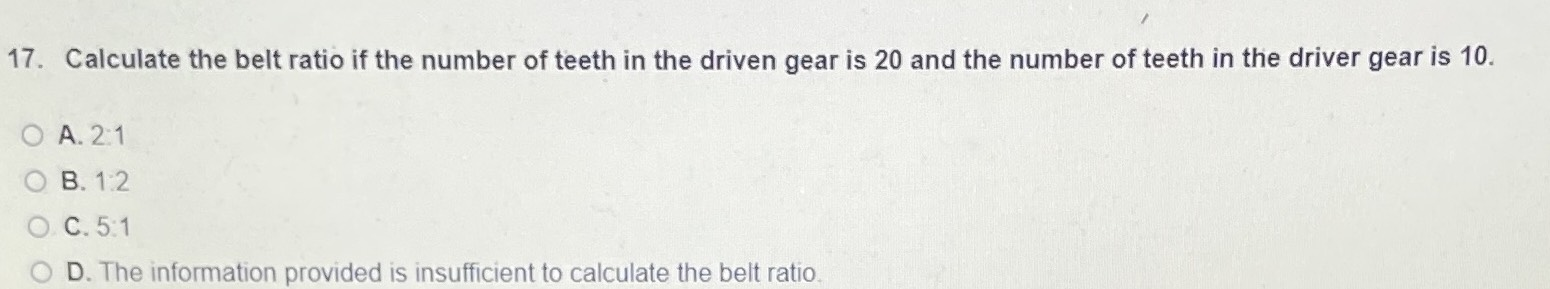### ¿Todavía tienes preguntas de matemáticas?

Pregunte a nuestros tutores expertos
Algebra
Pregunta17. Calculate the belt ratio if the number of teeth in the driven gear is $$20$$ and the number of teeth in the driver gear is $$10$$ .

A. $$2 : 1$$

B. $$1 : 2$$

C. $$5 : 1$$

D. The information provided is insufficient to calculate the belt ratio.